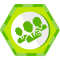## 如何计算时间复杂度 转

a*2^n+b*n^3+c*n^2+d*n*lg(n)+e*n+f

a ！ =0时，时间复杂度就是O(2^n);

a=0,b<>0 =>O(n^3);

a,b=0,c<>0 =>O(n^2)依此类推

eg:

(1)   for(i=1;i<=n;i++)   //循环了n*n次，当然是O(n^2)

for(j=1;j<=n;j++)

s++;

(2)   for(i=1;i<=n;i++)//循环了(n+n-1+n-2+...+1)≈(n^2)/2，因为时间复杂度是不考虑系数的，所以也是O(n^2)

for(j=i;j<=n;j++)

s++;

(3)   for(i=1;i<=n;i++)//循环了(1+2+3+...+n)≈(n^2)/2,当然也是O(n^2)

for(j=1;j<=i;j++)

s++;

(4)   i=1;k=0;

while(i<=n-1){

k+=10*i;

i++;      }

//循环了

n-1≈n次，所以是O(n)

(5)   for(i=1;i<=n;i++)

for(j=1;j<=i;j++)

for(k=1;k<=j;k++)

x=x+1;

//

log(a,b)=log(c,b)/log(c,a)

1.一个算法执行所耗费的时间，从理论上是不能算出来的，必须上机运行测试才能知道。但我们不可能也没有必要对每个算法都上机测试，只需知道哪个算法花费的时间多，哪个算法花费的时间少就可以了。并且一个算法花费的时间与算法中语句的执行次数成正比例，哪个算法中语句执行次数多，它花费时间就多。

2.一般情况下，算法的基本操作重复执行的次数是模块n的某一个函数fn），因此，算法的时间复杂度记做：Tn=Ofn））。随着模块n的增大，算法执行的时间的增长率和fn）的增长率成正比，所以fn）越小，算法的时间复杂度越低，算法的效率越高。

3.常见的时间复杂度

1.O(n)，O(n^2)， 立方阶O(n^3),... k次方阶O(n^k) 为多项式阶时间复杂度，分别称为一阶时间复杂度，二阶时间复杂度。。。。

2.O(2^n)，指数阶时间复杂度，该种不实用

3.对数阶O(log2n),   线性对数阶O(nlog2n)，除了常数阶以外，该种效率最高

for（i=1;i<=n;++i

{

for(j=1;j<=n;++j)

{

c[ i ][ j ]=0; //该步骤属于基本操作 执行次数：n^2

for(k=1;k<=n;++k)

c[ i ][ j ]+=a[ i ][ k ]*b[ k ][ j ]; //该步骤属于基本操作 执行次数：n^3

}

}

则有 Tn= n^2+n^3，根据上面括号里的同数量级，我们可以确定 n^3Tn）的同数量级

则有fn= n^3，然后根据Tn/fn）求极限可得到常数c

则该算法的 时间复杂度：Tn=On^3)

 定义：如果一个问题的规模是n，解这一问题的某一算法所需要的时间为T(n)，它是n的某一函数 T(n)称为这一算法的“时间复杂性”。 当输入量n逐渐加大时，时间复杂性的极限情形称为算法的“渐近时间复杂性”。 我们常用大O表示法表示时间复杂性，注意它是某一个算法的时间复杂性。大O表示只是说有上界，由定义如果f(n)=O(n)，那显然成立f(n)=O(n^2)，它给你一个上界，但并不是上确界，但人们在表示的时候一般都习惯表示前者。 此外，一个问题本身也有它的复杂性，如果某个算法的复杂性到达了这个问题复杂性的下界，那就称这样的算法是最佳算法。 “大O记法”：在这种描述中使用的基本参数是 n，即问题实例的规模，把复杂性或运行时间表达为n的函数。这里的“O”表示量级 (order)，比如说“二分检索是 O(logn)的”,也就是说它需要“通过logn量级的步骤去检索一个规模为n的数组”记法 O ( f(n) )表示当 n增大时，运行时间至多将以正比于 f(n)的速度增长。 这种渐进估计对算法的理论分析和大致比较是非常有价值的，但在实践中细节也可能造成差异。例如，一个低附加代价的O(n2)算法在n较小的情况下可能比一个高附加代价的 O(nlogn)算法运行得更快。当然，随着n足够大以后，具有较慢上升函数的算法必然工作得更快。 O(1) Temp=i;i=j;j=temp;                     以上三条单个语句的频度均为1，该程序段的执行时间是一个与问题规模n无关的常数。算法的时间复杂度为常数阶，记作T(n)=O(1)。如果算法的执行时间不随着问题规模n的增加而增长，即使算法中有上千条语句，其执行时间也不过是一个较大的常数。此类算法的时间复杂度是O(1)。 O(n^2) 2.1. 交换i和j的内容      sum=0；                 （一次）      for(i=1;i<=n;i++)       （n次 ）         for(j=1;j<=n;j++) （n^2次 ）          sum++；       （n^2次 ） 解：T(n)=2n^2+n+1 =O(n^2) 2.2.        for (i=1;i### 浮躁的码农2017/01/09
0
0

RichardBillion
01/06
0
0
1.数据结构&算法的引言+时间复杂度

print(sumOfN(10)) print(foo(10)) start_time = time.time()for a in range(0,1001): end_time = time.time()print(endtime-starttime) 执行结果为： 0 500 500200 375 425375 200 425500 0......

06/01
0
0
JavaScript 算法之最好、最坏时间复杂度分析

snowLu
01/07
0
0

2016/11/30
23
0

import android.content.Intent;import android.graphics.Bitmap;import android.os.Bundle;import android.os.Environment;import android.provider.MediaStore;import andr......

MrLins
45分钟前
4
0

47分钟前
7
0

Shingfi
49分钟前
4
0

DustinChan
55分钟前
6
0
Excel插入批注：可在批注插入文字、形状、图片

1.批注一直显示：审阅选项卡-------->勾选显示批注选项： 2.插入批注快捷键：Shift+F2 组合键 3.在批注中插入图片：鼠标右键点击批注框的小圆点【重点不可以在批注文本框内点击】----->调出批...

6
1